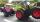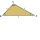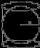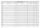# Basic functions - math word problems

1. Repair pipe20 workers had to repair broken pipes in 30 days. After fourteen days, four laborers joined them. How long did the pipe repair work last?
2. Land areaA land area of Asia and Africa are in a 3: 2 ratio, the European and African are is 1:3. What are the proportions of Asia, Africa, and Europe?
3. Ratio of edgesThe dimensions of the cuboid are in a ratio 3: 1: 2. The body diagonal has a length of 28 cm. Find the volume of a cuboid.
4. ApplesSchool kitchen bought 36kg apples for 12 kc/kg. How many kilograms of apples 1/4 cheaper can they buy for the same money?
5. Quarter circleWhat is the radius of a circle inscribed in the quarter circle with a radius of 100 cm?
6. TractorsTwo tractors plow the field in 4 hours together. If the first tractor plow half of the field and then the second tractor completed the job, it would take 9 hours. How many hours does the field plow for each tractor separately?
7. Evaluate expressionEvaluate expression using BODMAS rule: 1 1/4+1 1/5÷3/5-5/8
8. TrianglesFive sticks with a length of 2,3,4,5,6 cm. How many ways can you choose three sticks to form three sides of a triangle?
9. Stones in aquariumIn an aquarium with a length of 2 m, 1.5 m wide and 2.5 m deep, the water is up to three-quarters of the depth. Can we place 2m cubic meters of stones in the aquarium without spilling water? (0 = no, 1 = yes)
10. The thirdThe one-third rod is blue, one-half of the rod is red, the rest of the rod is white and measures 8 cm. How long is the whole rod?
11. Sequence 11What is the nth term of this sequence 1,1/2,1/3,1/4,1/5 ?
12. Equilateral cylinderA sphere is inserted into the rotating equilateral cylinder (touching the bases and the shell). Prove that the cylinder has both a volume and a surface half larger than an inscribed sphere.
13. The workThe work was to be done by 150 workers. At the beginning of their work, their number reduced by 40, which increased the time of work by 5 and 1/3 of the schedule. How long did work take?
14. ABCD squareIn the ABCD square, the X point lies on the diagonal AC. The length of the XC is three times the length of the AX segment. Point S is the center of the AB side. The length of the AB side is 1 cm. What is the length of the XS segment?
15. PerformanceTwo masons with the same performance would have made of plaster for 6 days. One of them, however, has increased its daily performance by 50%. How long would take they now to make plaster together?
16. Equilateral triangleA square is inscribed into an equilateral triangle with a side of 10 cm. Calculate the length of the square side.
17. 3 children3 children had to divide 4 pounds is candy. How much candy did each child get?
18. Parcel 4To send a parcel by messenger within city limits costs 60 cents for the first pound and 48 cents for each additional pound. What is the cost, in cents, of sending a parcel weighing p=4 pounds?
19. Cube, cuboid, and sphereVolumes of a cube and a cuboid are in ratio 3: 2. Volumes of sphere and cuboid are in ratio 1: 3. In what ratio are the volumes of cube, cuboid, and sphere?
20. Class alphabetAll 29 pupils in the class are written in a class by alphabet. The number of pupils enrolled before Paul is three times higher than the number of pupils behind him. Calculate how many pupils are enrolled after Paul.

Do you have an interesting mathematical word problem that you can't solve it? Enter it, and we can try to solve it.

To this e-mail address, we will reply solution; solved examples are also published here. Please enter the e-mail correctly and check whether you don't have a full mailbox.

Please do not submit problems from current active competitions such as Mathematical Olympiad, correspondence seminars etc...